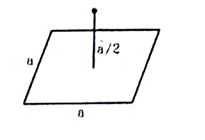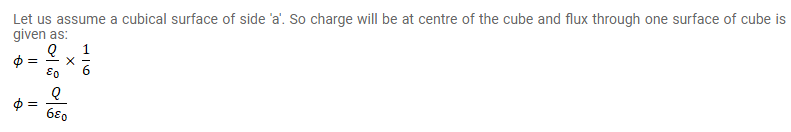# A charge Q is placed at a distance a/2 above the centre of a horizontal,Question:

A charge $\mathrm{Q}$ is placed at a distance $\mathrm{a} / 2$ above the centre of a horizontal, square surface of edge $\alpha$ as shown in figure. Find the flux of the electric field through the square surface.Solution: